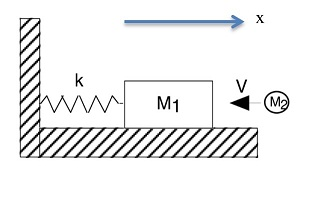+1-415-670-9189
info@expertsmind.com

# Get Solution

Write the equation of motion for the system
Course:- Physics
Reference No.:- EM13986541

 TweetExpertsmind Rated 4.9 / 5 based on 47215 reviews.
Review Site
Assignment Help >> Physics

A block of mass M1 = 5 kg is attached to a spring with a spring constant k = 20 N/m and rests on a frictionless horizontal surface. A wad of clay with mass M2 = 2 kg traveling at a speed of v = 14 m/s hits and sticks to the block.Find the frequency and amplitude of the subsequent simple harmonic oscillations.

Write the equation of motion for the system.

Draw a graph for the displacement as a function of time for this mass-spring system. Label the axes and scale the drawing accurately to show the period and amplitude.

How much time does it take for the spring to compress x = 1.5 meters from equilibrium for the first time?

Minimize